# Gate Mock Test- 6: CS/IT

## 65 Questions MCQ Test GATE Computer Science Engineering(CSE) 2023 Mock Test Series | Gate Mock Test- 6: CS/IT

Description
Attempt Gate Mock Test- 6: CS/IT | 65 questions in 180 minutes | Mock test for GATE preparation | Free important questions MCQ to study GATE Computer Science Engineering(CSE) 2023 Mock Test Series for GATE Exam | Download free PDF with solutions
QUESTION: 1

### Select the pair that does not expresses a relationship similar to that expressed in the pair: Wheel: spokes

Solution:

The spokes are units which radiate of a wheel and make the wheel a complete entity. Similarly, fingers, tentacles and petals are integral units of hand, octopus and flower respectively. On the other hand, roots and leaves do not share this kind of relationship. Thus option 4 does not expresses a relationship similar to that expressed in the given pair.

*Answer can only contain numeric values
QUESTION: 2

### If a, b and c are three positive integers such that a and b are in the ratio 3:4 while b and c are in the ratio 2:1, then minimum integer value of a + b + c is _________

Solution:

Let a = 3x and b = 4x

Similarly b = 2y and c = y

∴ 4x = 2y ⇒ y = 2x

∴ c = 2x

Now a + b + c = 3x + 4x + 2x = 9x

So, the minimum integer value = 9

QUESTION: 3

### Reaching a place of appointment of Friday. I found that I was two days earlier than the scheduled day. If I had reached on the following Wednesday then how many days late would I have been?

Solution:

Friday → 2 days earlier

Therefore, scheduled day = Friday + 2 = Sunday

Sunday + 3 = Wednesday

Therefore, I would have been late by 3 days

QUESTION: 4

Which one of the following options is the closest in meaning to the word 'mitigate'?

Solution:
QUESTION: 5

Choose the most appropriate word(s) from the options given below to complete the following sentence.

It was hoped at the time that that place would become the centre from which the civilization of Africa would proceed; but this ________ was not fulfilled.

Solution:

The sentence implies that it was hoped that that place would become the point from where the African civilization would proceed, but the belief that it would happen was not fulfilled.

Therefore, the correct word to fill in the blank is expectation as it means a strong belief that something will happen or be the case.

QUESTION: 6

3, k, 2, 8, m, 3

The arithmetic mean of the list of numbers above is 4. If k and m are integers and k ≠ m, what is the median of the list?

Solution:

Mean = 4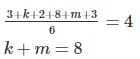k ≠ m so k = m = 4 is out.

{k, m} = {1, 7} or {2, 6} or {3, 5}

for median of {3, k, 2, 8, m, 3}

{1, 2, 3, 3, 7, 8} or {2, 2, 3, 3, 6, 8} or {2, 3, 3, 3, 5, 8}

Median: (3 + 3) /2 = 3

QUESTION: 7

Consider a random walk on an infinite two-dimensional triangular lattice, a part of which is shown in the figure below.If the probabilities of moving to any of the nearest neighbour sites are equal. What is the probability that the walker returns to the starting position at the end of exactly three steps?

Solution: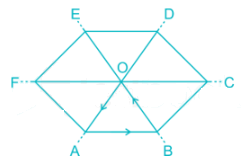A person can take 1st step in any direction independently

Suppose he moves to A

Now to return to O ( initial point) in 2 steps he can move in 2 directions. Either B or f

Thus probability he will move to either B or F will be = 2/6

Suppose he moves to B

Now to return back to O

he has only 1 option

⇒ to move in BO

Probability he will move in BO direction  = 1/6

Total probability he will return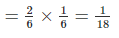QUESTION: 8

Twelve straight lines are drawn in a plane such that no two of them are parallel and no three of them are concurrent. A circle is now drawn in the same plane such that all the points of intersection of all the lines lie inside the circle. What is the number of non-overlapping regions into which the circle is divided?

Solution:

The nth line drawn will add n more regions to the circle.

1st line adds 1 region to the circle for a total of 2 regions.

2nd line adds 2 more regions to the circle, bringing the total number of regions to 2 + 2 = 4.

3rd line adds 3 more regions to the circle, bringing the total number of regions to 4 + 3 = 7.

4th line adds 4 more regions to the circle, bringing the total number of regions to 7 + 4 = 11.

5th line adds 5 more regions to the circle, bringing the total number of regions to 11 + 5 = 16.

6th line adds 6 more regions to the circle, bringing the total number of regions to 16 + 6 = 22.

7th line adds 7 more regions to the circle, bringing the total number of regions to 22 + 7 = 29.

8th line adds 8 more regions to the circle, bringing the total number of regions to 29 + 8 = 37.

9th line adds 9 more regions to the circle, bringing the total number of regions to 37 + 9 = 46.

10th line adds 10 more regions to the circle, bringing the total number of regions to 46 + 10 = 56.

11th line adds 11 more regions to the circle, bringing the total number of regions to 56 + 11 = 67.

12th line adds 12 more regions to the circle, bringing the total number of regions to 67 + 12 = 79.

QUESTION: 9

Electromagnetic radiation is an insidious culprit. Once upon a time, the major concern around electromagnetic radiation was due to high tension wires which carry huge amounts of electricity to cities. Now, we even carry sources of this radiation with us as cell phones, laptops, tablets and other wireless devices. While the most acute exposures to harmful levels of electromagnetic radiation are immediately realized as burns, the health effects due to chronic or occupational exposure may not manifest effects for months or years.

Which of the following can be the viable solution for electromagnetic radiation reduction?

Solution:

The correct answer is option 2 i.e. To implement hardware protocols to minimize risks and reduce electromagnetic radiation production significantly.

The passage states about the electromagnetic radiations released from the devices and how they affect the individuals. Out of the given options, only option 2 states the possible solution which can be implemented to reduce electromagnetic radiation.

QUESTION: 10

In a mock exam, there were 3 sections. Out of all students, 60 students cleared the cut off in section 1, 50 students cleared the cutoff in section 2 and 56 students cleared the cut off in section 3. 20 students cleared the cutoff in section 1 and section 2, 16 cleared cut off in section 2 & section 3, 26 cleared the cut off in section 1 & section 3. The number of students who cleared cutoff of the only one section was equal & was 24 for each section. How many students cleared cut off all the three sections?

Solution:

Let the number of students be X who cleared cut off in all sections.

The number of students who cleared the cutoff in section 1 and section 2, only = 20 – X

The number of students who cleared the cutoff in section 2 and section 3, only = 16 – X

The number of students who cleared the cutoff in section 1 and section 3, only = 26 – X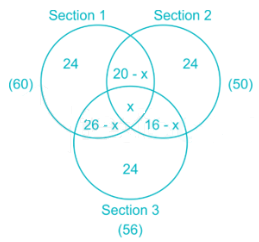Now, consider section 1:

24 + 20 – x + x + 26 – x = 60

70 – x = 60

x = 10

QUESTION: 11

The regular expression which represents the set of strings in which every 0 is immediately followed by at least two 1's is ____________.

Solution:

If w is in L, then either

(a) w does not contain any 0, or

(b) it contains a 0 followed by 11. So, w can be written as w1w2.........wn, where each wi is either 0 or 011.

So, L is represented by the regular expression (1 + 011)*.

Therefore, option 2 is the correct answer.

*Answer can only contain numeric values
QUESTION: 12

A and B are the only two host on a LAN which uses CSMA/CD protocol. A minimum time required by ‘A’ to detect collision is 600 μs. Find the time taken by the packet to travel from host A to host B.

Solution:

Concept:

Contention period is minimum time a host must transmit for before it can be sure that no other host's packet has collided with its transmission. It takes minimum of RTT to detect collision.

Calculation:

Contention Period = RTT = 2 × Tp

600 = 2 × Tp

Tp = 300 μs

*Answer can only contain numeric values
QUESTION: 13

What is the minterm that equals 1 if x1 = x3 = 0 and x2 = x4 = x5 = 1, and equals 0 otherwise?

Solution:

The minterm y1y2 ...yn is 1 if and only if each yi is 1, and this occurs if and only if xi = 1 when yi = xi and xi=0 when yi = xi.

Therefore, the minterm will be x1'x2x3'x4x5 i.e. 01011.

QUESTION: 14

Consider a 1024 MB free partition and the following memory request.

R1 requests 120 MB

R2 requests 250 MB

R3 requests 480 MB

R4 requests 40 MB

Which of the following allocation technique will merge the partition into the original 1024 KB segment, when R4 finishes?

Solution:

The buddy system allocates memory from a fixed-size segment consisting of physically contiguous pages. Memory is allocated from this segment using a power-of-2 allocator, which satisfies requests in units sized as a power of 2.

QUESTION: 15

Consider a double-ended queue with elements 31, 17, 4, 22, 19, 8. What is the time complexity of deletion of last element i.e. 8 and insertion of new element 10 at the rear?

Solution:

In a double-ended queue, elements can be inserted and deleted from both the front and back of the queue.

Time complexity of all operations like insert element at front, insert at rear, delete element from front, and delete element from rear is O(1).

QUESTION: 16

The column vector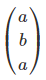is a simultaneous Eigen vector of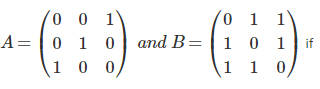Solution: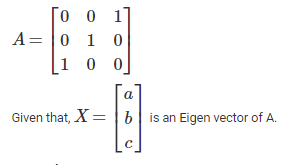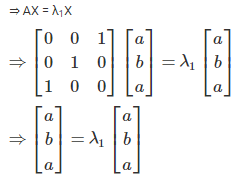For all values of a and b,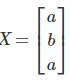is an Eigen vector of A.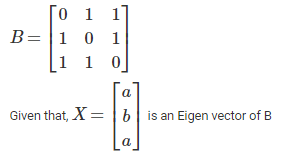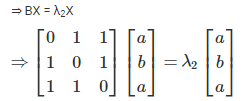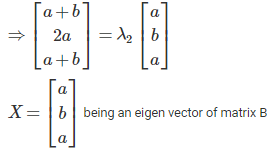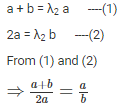⇒ ab + b2 = 2a2

⇒ 2a2 - ab - b2 = 0

⇒ 2a2 - 2ab + ab - b2 = 0

⇒ 2a (a - b) + b (a - b) = 0

⇒ b = a, b = -2a

For b = a, (or) b = -2a, X is an eigen vector of matrix B.

*Answer can only contain numeric values
QUESTION: 17

Consider a processor that includes a base with indexing addressing mode. Suppose an instruction is encountered that employs this addressing mode and specifies a displacement of 1500. Base register contains the value 3456 and index register contains the value 4. What is the address of the operand?

Solution:

In indexing addressing mode, the address field references a main memory address, and the referenced register contains a positive displacement from that address.

Address of operand = base register + index register + displacement

Address of operand = 3456 + 4 + 1500 = 4960

*Answer can only contain numeric values
QUESTION: 18

The address of a class C host is to be split into subnets with a n-bit subnet number. The maximum number of hosts in each subnet is 14. Find the value of n.

Solution:

The number of available hosts on a subnet is 2h−2, where h is the number of bits used for the host portion of the address.

h2 = 14

h = 4

QUESTION: 19

Which of the following options is INCORRECT?

Solution:

Option 4 is incorrect. It can be corrected as:

The cartesian product of two countable sets is countable.

Proof: There are three cases to consider:

Case 1: If both A and B are finite with |A|=m and |B|=n, then it is easy to show that |A×B|=mn and hence A×B is finite and so it is countable.

Case 2: If A is finite with |A|=n and B is countably infinite, there exist bijective functions f: A→{1,2,...,n} and g: B→N. We can define h: A×B→N for all (a,b)∈A×B.

Then clearly h is injective. So A×B is countable.

Case 3: If A and B are both countable, there exist bijective functions f: A→N and g: B→N and defining h: A×B→N gives us an injective function, and so A×B is countable.

QUESTION: 20

Consider a following resource allocation graph with multiple instance of each resource type.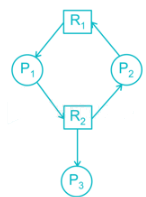Which of the following statement is true about above system?

Solution:

In resource allocation graph, a claim edge Pi → Rj indicates that process Pi may request resource Rj at some time in the future. An assignment edge Rj → Pi indicates that process Pi is holding resource Rj. The above system is deadlock-free although there is a cycle in a graph because there are more than one instance per resource type.

QUESTION: 21

Which of the following is the correct way to allocate a memory for the following structure?

struct Student

{

int sid;

int age;

};

Solution:

The sizeof operator returns the size of the struct in bytes. The size of a struct is not always the sum of the size of the individual members, hence use sizeof rather than a literal.

struct Student *sptr;

sptr = (struct Student*)malloc( sizeof(struct Student) );

QUESTION: 22

Solve the integral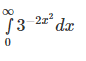Solution: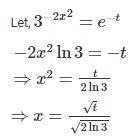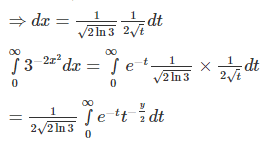We know that,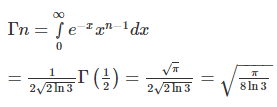*Answer can only contain numeric values
QUESTION: 23

In TCP connection, When SYN segment is sent, the value of retransmission-timeout is set to 11 sec. and when the SYN + ACK segment is received at the sender side the time required for the segment to reach the destination (i.e. at the sender side) and be acknowledged is equal to 3.2 sec. Find the retransmission – timeout.

Solution:

Concept:

The measured round-trip time for a segment is the time required for the segment to reach the destination and be acknowledged, although the acknowledgment may include other segments. In TCP, there can be only one RTT measurement in progress at any time.

Calculation:

RTTM = 3.2

RTTS = 3.2

RTTD = RTTS/2 = 1.6

RTO = RTTS + 4 × RTTD = 9.6

QUESTION: 24

Consider the following syntax directed definition: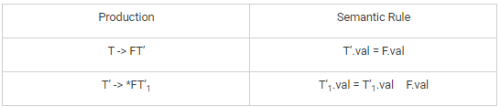The above SDD is

Solution:

The first rule defines the inherited attribute T’.val using F’.val and F appears to the left of T’ in the production. The second rule define T’1.val using the inherited attribute T’.val associated with head and F.val, where F appears to the left of T’ in the production

QUESTION: 25

There are two boxes each containing two components. Each component is defective with probability ¼, independent of all other components. The probability that exactly one box contains exactly one defective component equals?

Solution:

Probability (1 faulty in box)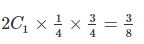The chances of box having other than 1 defective is 5/8

The probability exactly 1 one fault in exactly 1 box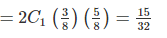Alternate:

The given situation is possible if

(1) 1 faulty among 4 components

(2) 3 faulty among 4 components

For case (1)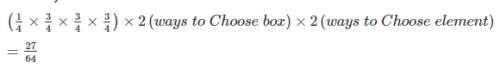For case (2)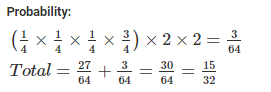QUESTION: 26

Which of the following statements is false?

Solution:

Out of all the options, option 3 is false.

It can be corrected as: It is desirable to maximize CPU utilization and throughput and to minimize turnaround time, waiting time, and response time.

*Answer can only contain numeric values
QUESTION: 27

A data is sent to UDP along with a pair of socket address and the length of data. After receiving data, UDP adds the header and passes the user datagram to IP with the socket address. What is the maximum size of the data that can be encapsulated in a UDP datagram?

Solution:

In IPv4, the maximum length of packet size is 65,536 and length of IP header is 20 bytes. So, maximum data size (including UDP header) = 65,535 – 20 = 65515

Size of UDP header = 8 bytes

Maximum data size = 65,515 – 8 = 65507

QUESTION: 28

What is the output of following C code:

#include <stdio.h>

int main(void) {

char *p;

p = "Programming";

p++ ;

++p;

--p;

p--;

printf( p );

return 0;

}

Solution: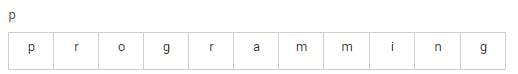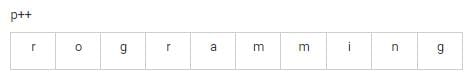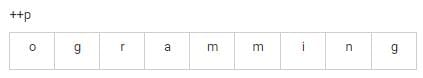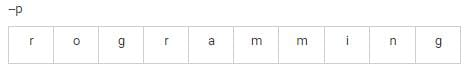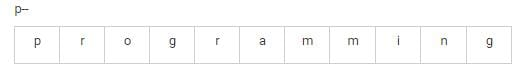*Answer can only contain numeric values
QUESTION: 29

f the number of balanced parenthesis possible with 'n' pair of parenthesis is 14, what is the value of 'n'?

Solution:

Number of balanced parenthesis = Number of binary search trees

Number of binary search trees =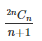When n = 4

Number of binary search trees =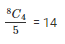Therefore, value of n = 4

*Answer can only contain numeric values
QUESTION: 30

Consider the following statements:

S1: The identifying relationship is many-to-one from the weak entity set to the identifying entity set, and the participation of the weak entity set in the relationship is total.

S2: It is possible to have a weak entity set with more than one identifying entity set.

The number of correct statements are ______

Solution:

Both the given statements are true.

It is possible to have a weak entity set with more than one identifying entity set. A particular weak entity would then be identiﬁed by a combination of entities, one from each identifying entity set. The primary key of the weak entity set would consist of the union of the primary keys of the identifying entity sets, plus the discriminator of the weak entity set.

QUESTION: 31

Consider the following statements:

A: The set of all reflexive relations are closed under the operation of set union.

B: The set of all irreflexive relations are not closed under the operation of set union.

C: The set of all reflexive relations are not closed under set difference.

D: The set difference of all reflexive relations is not irreflexive.

Which of the given statements are false?

Solution:

Statements B and D are incorrect. They can be corrected as:

• The set of all irreflexive relations are closed under the operation of the set union.
• The set difference of all reflexive relations is irreflexive.

Hence, option 1 is correct.

QUESTION: 32

Match the following: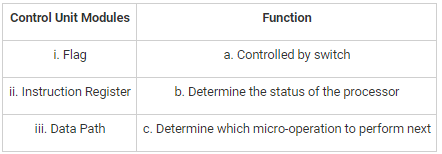Solution:

Flags: These are needed by the control unit to determine the status of the processor and the outcome of previous ALU operations.

Instruction register: The opcode and addressing mode of the current instruction are used to determine which micro-operations to perform during the execute cycle.

Data paths: The control unit controls the internal flow of data. For example, on instruction fetch, the contents of the memory buffer register are transferred to the instruction register. For each path to be controlled, there is a switch.

QUESTION: 33

Which of the following relations gives chromatic partition between vertices of the same colour in a connected graph?

Solution:

Equivalence relation between vertices of the same colour in a connected graph gives the chromatic partition.

*Answer can only contain numeric values
QUESTION: 34

Consider the minterm list form of a Boolean function F given below:

F(P, Q, R, S) = Ʃm(0, 1, 2, 4, 6, 8, 9, 10) + d(3, 11, 15)

Here, m denotes a minterm and d denotes a don't care term. The number of essential prime implicants of the function F is ___________.

Solution: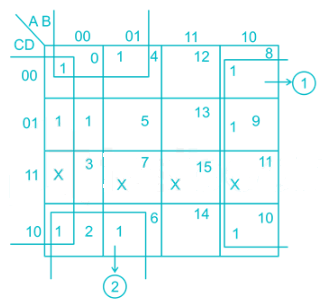Therefore, the number of essential prime implicants is 2.

*Answer can only contain numeric values
QUESTION: 35

Consider a relational schema R(A, B, C, D, E, F, G) with functional dependencies:

A → BC

C → DG

C → E

D → FG

The number of superkeys possible is _____.

Solution:

The candidate key for the given schema is A because (A)+ = {A, B, C, D, E, F, G, H}

Note: When there is only candidate key for a relation with n-attributes, there are 2n-1 super keys possible.

In the given relation, there are 7 attributes. Therefore, the total number of super keys possible are 26 = 64.

QUESTION: 36

Match the RAID levels with the number of disks required.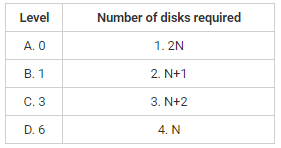Solution:

The different RAID levels with the number of disks required are as follows: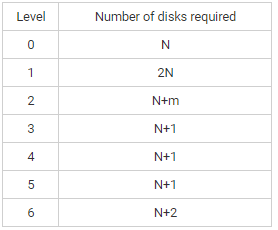where N = number of data disks and m is proportional to logN.

*Answer can only contain numeric values
QUESTION: 37

Consider a software program that is artificially seeded with 100 faults. While testing this program, 159 faults are detected, out of which 75 faults are from those artificially seeded faults. Assuming that both real and seeded faults are of same nature and have same distribution, the estimated number of undetected real faults is________.

Solution:

As both real and artificial faults have the same distribution, they will be detected in same proportions.

≠ A.F. = 100

≠ R.F. = x

A fraction of faults detected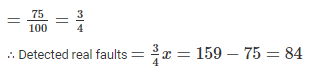*Answer can only contain numeric values
QUESTION: 38

Consider the following C function:

int foo()

{

int i;

int sum = 0;

for(i = 1; i < = 20; i++)

sum = sum + i;

return sum;

}

Calculate the least number of temporary variables required to create an intermediate code for above C function.

Solution:

The intermediate code for above code:

1. sum = 0;

2. i = 0;

3. if(i > 10) goto

4. t1 = sum + i;

5. sum = t1

6. t2 = i + 1;

7. i = t2;

8. goto 3

9. goto calling program

QUESTION: 39

Who invented OOP?

Solution:

Alan Kay invented OOP, Andrea Ferro was a part of SmallTalk Development. Dennis invented C++ and Adele Goldberg was in team to develop SmallTalk but Alan actually had got rewarded for OOP.

*Answer can only contain numeric values
QUESTION: 40

A host machine uses the token bucket for congestion control with a capacity of 9 GB and the maximum output rate is 250 MBps. The minimum time required to transmit the data is 50 seconds. Tokens arrive to sustain output at a rate of x MBps per second. Find the value of x.

Solution:

C = capacity of bucket = 1 GB

M = maximum output rate = 250 MBps

e = Input rate​

S = minimum time required to transmit the data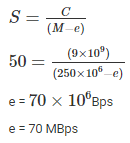The output rate is 250 MBps. Tokens arrive at a rate to sustain output at a rate of x MBps.

M – e = 250 – 70 = 180 MBps

*Answer can only contain numeric values
QUESTION: 41

Let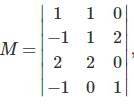, then the rank of M is equal to

Solution:

Solution:

R2 → R2 + R1

R4 → R+ R1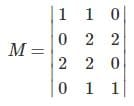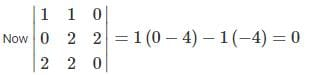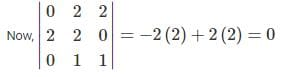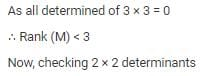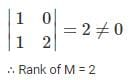QUESTION: 42

There are 15 printers. The current allocation and maximum requirement of tape drives for four processes are shown below: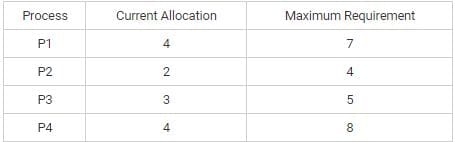Which of the following is true as per the current state of the system?

Solution:

The system is in safe and non deadlocked state. The safe sequence is P2 -> P3 -> P4 -> P1.

*Answer can only contain numeric values
QUESTION: 43

In a class of 15 students a quiz is held. The sum of their scores is 100. How many students atleast must have the same score?

Solution:

Suppose the scores are all different.

Therefore, we can arrange them in order s1< s2< ...< s15. The smallest possible values here would be s1=0, s2=1, s3=2, ..., s15=14.

If we add these scores, we get (14)(15)/2 = 105. This is a contradiction since the scores are supposed to sum to 100.

Thus, the scores cannot be all different. Atleast 2 students must have the same score.

*Answer can only contain numeric values
QUESTION: 44

Consider the following undirected weighted graph.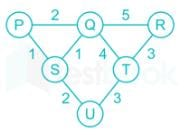Find the minimum possible weight of the spanning tree if Kruskal’s algorithm is implemented on the above graph.

Solution:

Above graph contains 6 vertices, so minimum spanning tree will be having 6 – 1 = 7 edges. Sort the edges in increasing order of their weight.

Pick the smallest edge. Check if it forms a cycle with the spanning tree formed so far. If the cycle is not formed, include this edge. Else, discard it. Repeat these steps until there are 7 edges in the MST.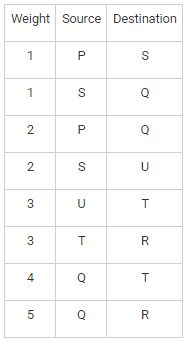MST will contain edge (P,S), (S,Q), (S,U), (U,T), (T,R).

Hence, minimum possible weight = 1 + 1 + 2 + 3 + 3 = 10

QUESTION: 45

What is the count of the non-zero entries in a triangular matrix?

Solution:

We know that in a lower triangular matrix, the non-zero entries occur only on or below the main diagonal.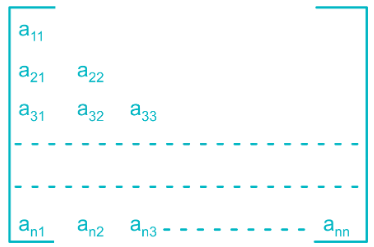Therefore, the elements are stored in a linear array M in the following way:

M = a11

M = a21

M = a22

M = a31

and so on.

It can be observed that A contains 1 element in the first row, 2 elements in the second row, 3 elements in the third row and likewise n elements in the nth row.

Therefore, the total number of elements that M will contain = 1+2+3+...+n=n(n+1)/2

*Answer can only contain numeric values
QUESTION: 46

What is the predicted value of the fifth CPU burst (in µsec) if exponential averaging is used in the case of the shortest job first scheduling algorithm? The length of CPU bursts µsec is {t1, t2, t3, t4} = {4, 5, 8, 7}, smoothening factor is 0.6 and predicted value of the first CPU burst is 8 µsec.

Solution:
QUESTION: 47

Consider the following Turing machine: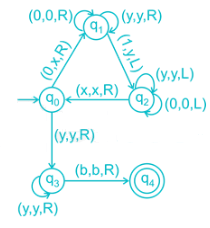Note: (p, q, r) represents that by reading input 'p', it replaces 'p' by 'q' and moves to 'r' direction. Which of the following languages is accepted by the above Turing machine?

Solution:

The given Turing machine performs the following:

(a) If the leftmost symbol in the given input string w is 0, it is replaced by x and moved right till a leftmost 1 is encountered in w. It is changed to 'y' and moved backwards.

(b) Step (a) is repeated with the leftmost 0. When no 0 or 1 is left, it is moved to a final state.

Therefore, the sequence which is printed is of the form 0n1n.

Hence, option 1 is the correct answer.

*Answer can only contain numeric values
QUESTION: 48

Consider the following x86 machine instruction sequence:

SUB ECX, EAX

The first instruction adds the contents of the 32-bit registers EAX and EBX and stores the result in EAX. The second instruction subtracts the contents of EAX from ECX and stores the result in ECX. These instructions are to be executed in a pipelined instruction processor with the following 4 stages: fetch instruction (FI), decode instruction and calculate addresses (DA), fetch operand (FO), and execute (EX). The FI, DA and EX stages take 1 clock cycle each for any instruction. The FO stage takes 2 clock cycle for ADD and 3 clock cycle for SUB instruction. The pipelined processor uses operand forwarding from the FO stage to the DA stage. Calculate the number of clock cycles required for the execution of the above instructions.

Solution: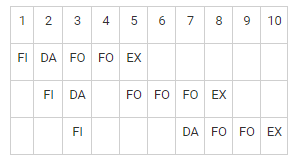*Answer can only contain numeric values
QUESTION: 49

The minimum number of states in the NFA for the regular expression (a + a(b + aa)*b)* a(b + aa)* a is ______.

Solution:

The NFA for the given regular expression is: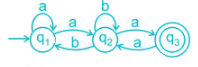Therefore, the number of states = 3

*Answer can only contain numeric values
QUESTION: 50

Assume that the main memory with only 4 page frames which are initially empty. If the page reference string is a b c d a e f b c d c e d b f, the number of page faults using the optimal page replacement policy is ______.

Solution:
*Answer can only contain numeric values
QUESTION: 51

Consider a simple graph with 10 vertices. The graph will be a connected graph if it has atleast _______ edges

Solution:

Note: The simple graph with n-vertices is connected if it has atleast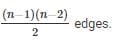Therefore, number of edges = 9*8/2 = 36

QUESTION: 52

Consider the following graph. If DFS is implemented on the following graph then which of the following node will not be marked as visited at the end of the traversing if search is started at node A?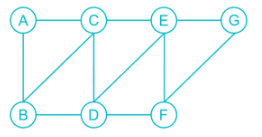Solution:

Some of the sequences of the node in the order they are first visited:

ABCDEFG

ACEGFDB

ABDFGEC

ACBDEGF

ACBDFGE

ABCEGFD

QUESTION: 53

Solution:

IP address → 165.81.35.120 → 10100101 01010001 00100011 01111000

Subnet mask → 255.255.192.0 → 11111111 11111111 11000000 00000000

IP address                   10100101 01010001 00100011 01111000

Subnet mask               11111111 11111111 11000000 00000000

Subnet address           10100101 01010001 00000000 00000000

Subnet address → 10100101 01010001 00000000 00000000 → 165.81.0.0

QUESTION: 54

Consider the following statements about the dining philosopher problem

I. There should be at least 6 chopsticks to avoid deadlock for 6 philosophers.

II. If the asymmetric solution is implemented then 1stphilosopher picks up her right chopstick first while 6thphilosopher picks up her left chopstick first.

Which of the above statement is correct?

Solution:

I. There should be at least n + 1 chopsticks to avoid deadlock for n philosophers.

II. Asymmetric solution: an odd philosopher picks up first her left chopstick and then her right chopstick, whereas an even philosopher picks up her right chopstick and then her left chopstick.

QUESTION: 55

Consider the new order traversal of a binary tree:

• Visit right sub-tree using new order traversal.
• Visit root.
• Visit left sub-tree using new order traversal.

The post-order traversal of a binary tree is 3, 2, 3, 5, +, ↑, *, 1, -. What is the new order traversal of the same tree?

Solution:

In post-order traversal, the left subtree is traversed first, then the right subtree and finally the root node.

According to the given post-order traversal, the root node should be '-' because it comes at the last in the expression. Similarly, 3 should be the leftmost node of the tree because it is at the starting of the expression. This process is continued and the resulting tree is obtained.

The equivalent expression tree for the given post-order traversal is: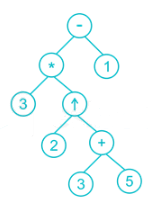Therefore, the new order traversal is: 1, -, 5, +, 3, ↑, 2, *, 3.

QUESTION: 56

Consider the following tuple relational calculus: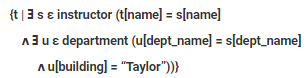What does the given expression perform?

Solution:

The correct answer is option 2.

There are two 'there exists' clauses in the given tuple relational calculus which are connected by and (ᴧ). The tuple variable u is restricted to departments that are located in the Taylor building, while tuple variable s is restricted to instructors whose dept name matches that of tuple variable u.

Therefore, the given expression finds the names of all instructors whose department is in the Taylor building.

QUESTION: 57

What is the output of the following C code:

#include<stdio.h>

void fun1()

{

auto int x = 1;

static int y;

register char z = 'F';

printf("%d %d %d", x, y, ++z);

}

int main()

{

fun1();

return 0;

}

Solution:

Static variable:

The value of a static variable persists until the end of the program.

Automatic variable:

The variables declared inside the function are automatic or local variables.

External variable:

Variables that are declared outside of all functions are known as external variables. External or global variables are accessible to any function.

Register variable:

The register storage class is used to define local variables that should be stored in a register instead of RAM.

QUESTION: 58

If the expression has k variables and n operator occurrences, what is the running time complexity to check whether the expression is tautology or not?

Solution:

If the expression has k variables and n operator occurrences, the table has 2k rows, and there are n columns that need to be filled out.

Therefore, the implementation of this algorithm takes O(2kn) time

*Answer can only contain numeric values
QUESTION: 59

Consider the following function. What is the output of calc(10, 1, 2) if pow(y, z) returns value of yz

int calc(int x, int y, int z)

{

int temp = pow(y, z);

if(temp == x) return 1;

if(temp > x) return 0;

return calc(x, y+1, z) + calc(x - temp, y+1, z);

}

Solution: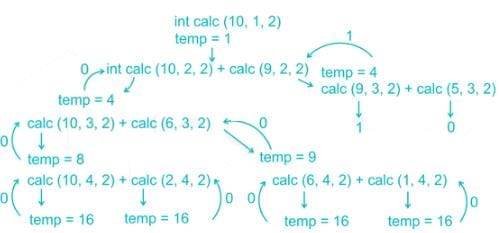*Answer can only contain numeric values
QUESTION: 60

Suppose a set S ={a1, a2, a3, …} of n proposed activities used by a person. Each activity ai has a start time si and a finish time fi , where 0 <= si < fi < ∞∞. Find the maximum number of activities that can be performed by a single person, assuming that a person can only work on a single activity at a time.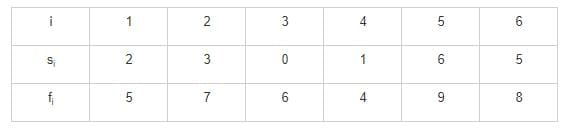Solution:

ort the activities according to their finishing time.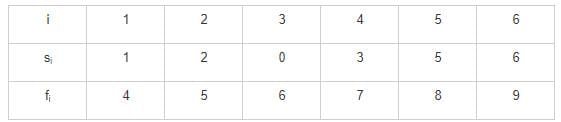Select the first activity from the sorted array

If the start time of this activity is greater than or equal to the finish time of a previously selected activity then select this activity.

Possible set of activities:

(1,4), (5,8)

(2,5), (5,8)

(0,6), (6,9)

QUESTION: 61

Consider the following set of statements:

A: The write-back policy increases memory writes.

B: Direct mapping technique faces the problem of thrashing.

C: Temporal locality refers to the tendency of execution to involve a number of memory locations that are clustered.

Which of the given statements is/are incorrect?

Solution:

Write back, minimizes memory writes. With write back, updates are made only in the cache. When an update occurs, a dirty bit, or use bit, associated with the line is set. Then, when a block is replaced, it is written back to main memory if and only if the dirty bit is set.

The main disadvantage of direct mapping is that there is a fixed cache location for any given block. Thus, if a program happens to reference words repeatedly from two different blocks that map into the same line, then the blocks will be continually swapped in the cache, and the hit ratio will be low (a phenomenon known as thrashing).

Spatial locality refers to the tendency of execution to involve a number of memory locations that are clustered. This reflects the tendency of a processor to access instructions sequentially. The spatial location also reflects the tendency of a program to access data locations sequentially, such as when processing a table of data.

Therefore, option 1 is the correct answer.

QUESTION: 62

Consider the following relations:

student(ID, name, dept_name, credits)

course(ID, course_ID, sec_ID, semester, year, grade)

Which of the following is the correct SQL for "For each course section offered in 2009, ﬁnd the average total credits of all students enrolled in the section, if the section had at least 2 students".

Solution:

The correct answer is option 2.

The sequence of operations is as follows:

• The from clause is ﬁrst evaluated to get a relation.
• The predicate in the where clause is applied to the result relation of the from clause.
• Tuples satisfying the where predicate are then placed into groups by the group by clause.
• The having clause is applied to each group; the groups that do not satisfy the having clause predicate are removed.
• The select clause uses the remaining groups to generate tuples of the result of the query, applying the aggregate functions to get a single result tuple for each group.

Satisfying the given sequence of operations, only option 2 performs the desired operation.

*Answer can only contain numeric values
QUESTION: 63

Consider the following graph: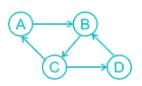What is the number of topological orders for the above graph?

Solution:

Topological sorting is a linear ordering of vertices such that for every directed edge uv, vertex u comes before v in the ordering. Topological Sorting for a graph is not possible if the graph is not a DAG i.e. if a cycle is present in the graph.

In the given graph, cycle is present. Therefore, no topological order is possible for the given graph.

*Answer can only contain numeric values
QUESTION: 64

Let A, B, C, and D be four matrices of dimensions 10 x 9, 9 x 12, 12 x 10, and 10 x 15, respectively. The minimum number of scalar multiplications required to find the product ABCD using the basic matrix multiplication method is ______.

Solution:

((AB)C)D = (10 x 9, 9 x 12), 12 x 10, 10 x 15

= 10 x 9 x 12 + 10 x 12 x 10 + 10 x 10 x 15

= 3780

A(BC)D =9 x 12 x 10 + 10 x 9 x 10 + 10 x 10 x 15

= 3480

A(B(CD)) = 12 x 10 x 15 + 9 x 12 x 15 + 10 x 9 x 15

= 4770

(AB)(CD) = 10 x 9 x 12 + 12 x 10 x 15 + 10 x 12 x 15

= 4680

*Answer can only contain numeric values
QUESTION: 65

What is the number of AND gates required in carry circuit for 10- bit look ahead carry adder?

Solution:

The number of AND gates required in carry circuit for a n-bit look ahead carry adder is given by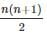Therefore, number of AND gates = 10*11/2 = 55Use Code STAYHOME200 and get INR 200 additional OFF Use Coupon Code